Courses

# Test: Discrete-Time Systems

## 15 Questions MCQ Test RRB JE for Electrical Engineering | Test: Discrete-Time Systems

Description
This mock test of Test: Discrete-Time Systems for Railways helps you for every Railways entrance exam. This contains 15 Multiple Choice Questions for Railways Test: Discrete-Time Systems (mcq) to study with solutions a complete question bank. The solved questions answers in this Test: Discrete-Time Systems quiz give you a good mix of easy questions and tough questions. Railways students definitely take this Test: Discrete-Time Systems exercise for a better result in the exam. You can find other Test: Discrete-Time Systems extra questions, long questions & short questions for Railways on EduRev as well by searching above.
QUESTION: 1

### x[n] and h[n] are given in the question. Compute the convolution y[n] = x[n] * h[n] and choose correct option. Que: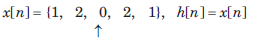Solution: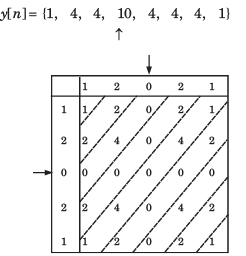QUESTION: 2

### x[n] and h[n] are given in the question. Compute the convolution y[n] = x[n] * h[n] and choose correct option. Que: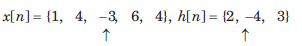Solution:QUESTION: 3

### x[n] and h[n] are given in the question. Compute the convolution y[n] = x[n] * h[n] and choose correct option.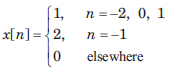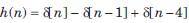Solution: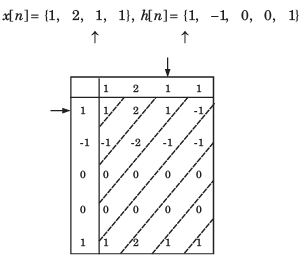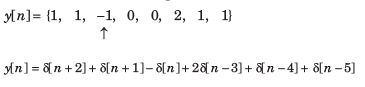QUESTION: 4

In question y[n] is the convolution of two signal. Choose correct option for y[n].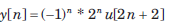Solution: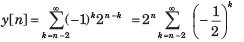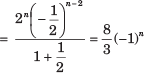QUESTION: 5

In question y[n] is the convolution of two signal. Choose correct option for y[n].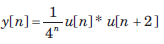Solution: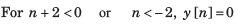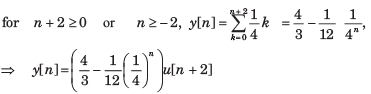QUESTION: 6

In question y[n] is the convolution of two signal. Choose correct option for y[n].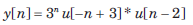Solution: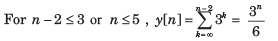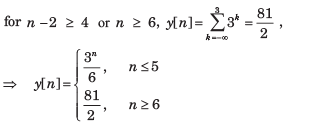QUESTION: 7

In question y[n] is the convolution of two signal. Choose correct option for y[n].

y[n] = u[n + 3] * u[n - 3]

Solution: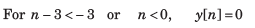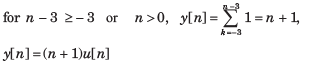QUESTION: 8

The convolution of x[n] = cos ( π/2 n) u[n] and h[n] = u[n - 1] is f [n]u[n - 1]. The function f [n] is

Solution: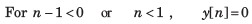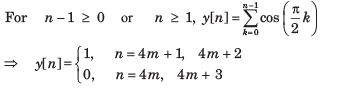QUESTION: 9

Let P be linearity, Q be time invariance, R be causality and S be stability. In question discrete time input x[n] and output y[n] relationship has been given. In the option properties of system has been given. Choose the option which match the properties for system.

Que: y[n] = rect (x[n])

Solution: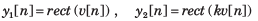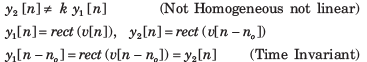At any discrete time n = no , the response depends only on the excitation at that discrete time. (Causal)

No matter what values the excitation may have the response can only have the values zero or one.

QUESTION: 10

Let P be linearity, Q be time invariance, R be causality and S be stability. In question discrete time input x[n] and output y[n] relationship has been given. In the option properties of system has been given. Choose the option which match the properties for system.

y[n] = nx[n]

Solution: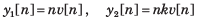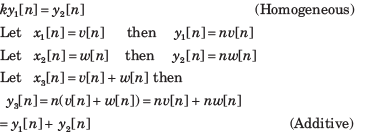Since the system is homogeneous and additive, it is also linear.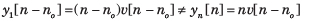At any discrete time, n = no the response depends only on the excitation at that same time. (Causal)

If the excitation is a constant, the response is unbounded as n approaches infinity. (Unstable)

QUESTION: 11

Let P be linearity, Q be time invariance, R be causality and S be stability. In question discrete time input x[n] and output y[n] relationship has been given. In the option properties of system has been given. Choose the option which match the properties for system.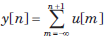Solution: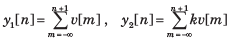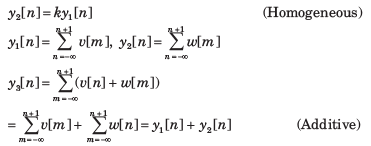Since the system is homogeneous and additive it is also linear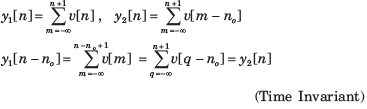At any discrete time, n =no , the response depends on the excitation at the next discrete time in future. (Anti causal)

If the excitation is a constant, the response increases without bound (Unstable)

QUESTION: 12

Let P be linearity, Q be time invariance, R be causality and S be stability. In question discrete time input x[n] and output y[n] relationship has been given. In the option properties of system has been given. Choose the option which match the properties for system.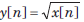Solution: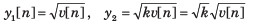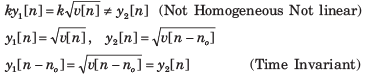At any discrete time n = no , the response depends only on the excitation at that time (Causal)

If the excitation is bounded, the response is bounded. (Stable).

QUESTION: 13

The output signal when a signal x(n)=(0,1,2,3) is processed through an ‘Delay’ system is:

Solution:

An delay system is a system whose output is same as the input, but after a delay.

QUESTION: 14

x[n] as shown in fig.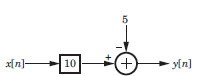Solution: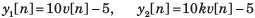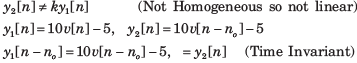At any discrete time, n = n0 the response depends only on the excitation at that discrete time and not on any future excitation. (Causal)

If the excitation is bounded, the response is bounded. (Stable).

QUESTION: 15

x[n] as shown in fig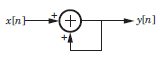Solution: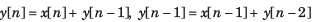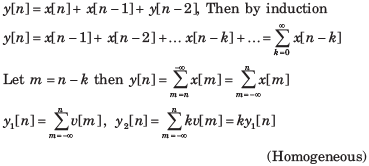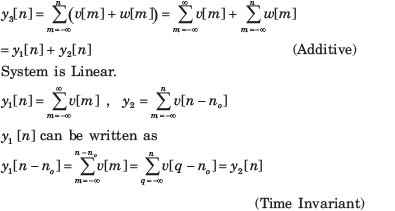At any discrete time n no the response depends only on the excitation at that discrete time and previous discrete time. (Causal)

If the excitation is constant, the response increase without bound. (Unstable)